# Dynamical systems software packages

software for dynamical systems

Mathematical background on dynamical systems can be found in [a3], [a7] or [a9] (cf. also Dynamical system). Numerical methods are described in [a3], [a6] and [a9].

In its basic form a dynamical system is a system of ordinary differential equations of the form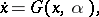(a1)

where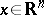is the state variable,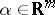is a parameter vector and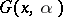is a non-linear function ofand. The independent variableis usually identified with time. The equilibria of (a1) are its constant solutions, i.e. the solutions of the non-linear system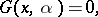(a2)

for a given parameter vector. Equilibria are asymptotically stable if all eigenvalues of the Jacobian matrix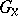have a strictly negative real part (cf. also Jacobi matrix). They are unstable if at least one eigenvalue has a strictly positive part. In the remaining cases the stability depends on the non-linear terms in the Taylor expansion of(cf. also Stability theory).

If a component ofis freed, then curves of equilibria can be computed. Generically, curves of equilibria can bifurcate in two ways (cf. also Bifurcation). The first is the limit point bifurcation, wherebecomes singular, i.e. has an eigenvalue zero (cf. also Limit point of a trajectory). Generically, this indicates a turning point of equilibria. If the equilibrium was initially stable, then it generically loses the stability.

The second bifurcation is the Hopf bifurcation, wherehas a conjugate pair of pure imaginary eigenvalues, i.e. with real part zero. Generically, a curve of periodic orbits is born in a Hopf point. If the equilibrium was initially stable, then generically it loses stability.

A periodic orbit is a solution of (a1) for which there exists a periodsuch that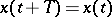for one and hence all values of. The linearized return mapping of a periodic orbit (cf. also Poincaré return map) is called the monodromy matrix. The eigenvalues of the monodromy matrix are the Floquet multipliers (cf. also Floquet exponents; Floquet theory). There is always one multiplier equal to. If all other multipliers have moduli strictly less than, then the periodic orbit is asymptotically stable. If at least one multiplier has modulus strictly larger than, then the periodic orbit is unstable. In the remaining cases the stability depends on the non-linear terms in the Taylor expansion of the return mapping.

Again, if a component ofis freed, then curves of periodic orbits can be computed.

The periodic orbits that originate at a Hopf point can be either stable or unstable. The stability is guaranteed if the equilibrium preceding to the Hopf point is stable and a quantity, called the first Lyapunov coefficient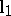, is negative (cf. also Lyapunov characteristic exponent). The bifurcation is then supercritical, i.e. the stable periodic orbits are found at the side where the equilibria are unstable. If the equilibrium preceding to the Hopf point is stable andis positive, then the periodic orbits are unstable and the bifurcation is subcritical, i.e., the periodic orbits are found at the side of the stable equilibria. The intermediate case where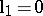is called a generalized Hopf or Bautin point.

The notion of a dynamical system can be extended in several ways. A discrete dynamical system is an iterated mapping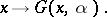(a3)

A delay differential equation is an equation of the form (a1) whereis also explicitly dependent on the values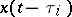for one or several delays(cf. also Differential equations, ordinary, retarded). It is a neutral differential equation ifis also explicitly dependent on the values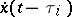for one or several delays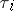(cf. also Neutral differential equation). A partial differential equation of evolution type is also considered as a dynamical system (cf. also Evolution equation).

## Software.

A website on dynamical systems software is [a8].

### AUTO.

The most widely used software package for dynamical systems computations is AUTO97 [a4]. This software is distributed freely; see [a1]. A manual is also available from this site. AUTO has many interesting features:

It can compute solution branches of (a2), detect and compute branch points and compute the bifurcating branches. It can also detect and compute limit points and Hopf points and continue these in two parameters. Also, it can find extrema of an objective function along solution branches and continue such extrema in more parameters.

It can compute fixed points for the discrete dynamical system (a3). It can compute branches of such fixed points, detect, compute and continue fold points, period-doubling (flip) and Neimark–Sacker bifurcations of fixed points.

It can perform a bifurcation analysis of (a1). It can compute branches of stable and unstable periodic orbits and compute the Floquet multipliers. Periodic orbits can be started from Hopf bifurcation points. Along branches of periodic orbits branch points, fold points, period-doubling, and torus bifurcations can be computed. In branch and period doubling bifurcations branch switching is possible.

Period-doubling bifurcations, folds, torus bifurcation points, and orbits with fixed period can be continued in two parameters.

It can follow curves of homoclinic orbits and detect and continue various codimension-homoclinic orbits.

It can locate extrema of an integral objective function along a branch of periodic solutions and continue such extrema in more parameters.

It can also compute curves of solutions to (a1) on a fixed intervalsubject to general non-linear integral and boundary conditions. Folds and branch points can be computed along such curves. Curves of folds can be computed and branch switching at branch points is provided.

It can further do some stationary and wave calculations for partial differential equations of the form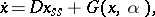(a4)

whereis a diagonal matrix of diffusion constants anddepends on timeand a one-dimensional space variable.

In AUTO, the numerical quality of the algorithms is strongly emphasised and the graphical user interface got less attention. In fact, AUTO can be used in command mode, i.e. without any graphical interface.

### CONTENT.

Another important package is CONTENT [a10], whose main developer is Yu.A. Kuznetsov.

CONTENT is a CONTinuation EnvironmeNT and the user interaction is via a windowing system. For algebraic equations (a2) as equilibrium solutions of (a1), CONTENT provides more routines than does AUTO. In fact, it allows one to detect all codimension-two bifurcations and to continue them numerically if a third parameter is freed. These codimension-two bifurcations are: Bogdanov–Takens, zero-Hopf, double Hopf, cusp, and generalized Hopf. The behaviour of dynamical systems near codimension-two equilibrium bifurcations is described in [a7] and [a9]. Generically, periodic orbits, homoclinic orbits, invariant tori, and chaotic behaviour can all be detected. CONTENT even allows one to detect and compute certain codimension-bifurcations, such as triple zero, swallowtail, resonant double Hopf, and a few others. Also, in most cases CONTENT offers several computational routines to compute and continue bifurcation points.

For discrete dynamical systems (a3), CONTENT offers the same possibilities as AUTO but leaves the user options to use several methods.

For dynamical systems (a1), CONTENT offers less routines than AUTO. However, it allows one to compute curves of periodic orbits and to detect the fold, flip and Neimark–Sacker bifurcations.

For partial differential equations, CONTENT allows a wider class of one-dimensional problems than does AUTO; actually, in (a4) the right-hand side can be replaced by practically any "reasonable" function and the boundary conditions can be quite general. On the other hand, only the time evolution computation of such systems is at present (2000) supported and only by the implicit Euler and Crank–Nicolson methods.

### Other packages.

A third roughly comparable package is CANDYS/QA (see [a8] for more information).

DsTool [a8] can compute equilibria of ordinary differential equations and diffeomorphisms and compute their stable and unstable manifolds. Several packages, notably DsTool, Dynamics Solver and XPP simulate and numerically solve dynamical systems equations. Several other packages, notably Global Manifolds 1D, Global Manifolds 2D, GAIO and BOV-method compute invariant manifolds. See [a8] for details.

For partial differential equations, the choice of software is limited. In addition to the capabilities of AUTO and CONTENT there is PDECONT [a8] for the continuation of periodic solutions of partial differential equations. Next, there exists the software package PLTMG [a2] that allows one to solve a whole class of boundary value problems on regions in the plane, to continue the solution with respect to a parameter, and even to compute branching points and limit points. This software combines a sophisticated finite-element discretization with advanced linear algebra techniques.

For delay differential equations there is the bifurcation package DDE-BIFTOOL [a5].